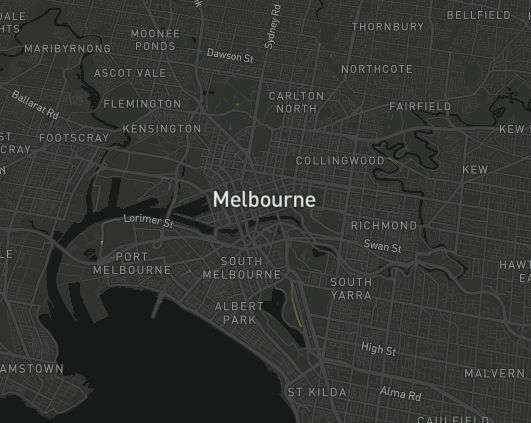The trips layer requires an sf object with XYZM dimension, where Z is elevation and M is a timestamp

In this example I’m taking the mapdeck::roads data, and assigning a random timestamp to each coordinate point in the lines.

The timestamps have to be positive, and in sequential order.

library(sf)
library(data.table)

set_token( "MAPBOX_TOKEN" )
setDT( dt )

dt[, elev := 0 ]
dt[, seq := 1:.N, by = linestring_id ]
dt1 <- dt[ seq == 1]
dt1[, start_time := sample(1:100, size = .N, replace = T)]
dt[
dt1[, .(linestring_id, start_time)]
, on = c("linestring_id")
, start_time := i.start_time
]

dt[, time := start_time + seq ]
s <- dt[, min( time )]
e <- dt[, max( time )]

setorder( dt, linestring_id )
obj = dt
, x = "x"
, y = "y"
, z = "elev"
, m = "time"
, linestring_id = "linestring_id"
)

sf\$geometry <- sfc
mapdeck(
location = c(145, -37.8)
, zoom = 10
, style = mapdeck_style("dark")
) %>%
)